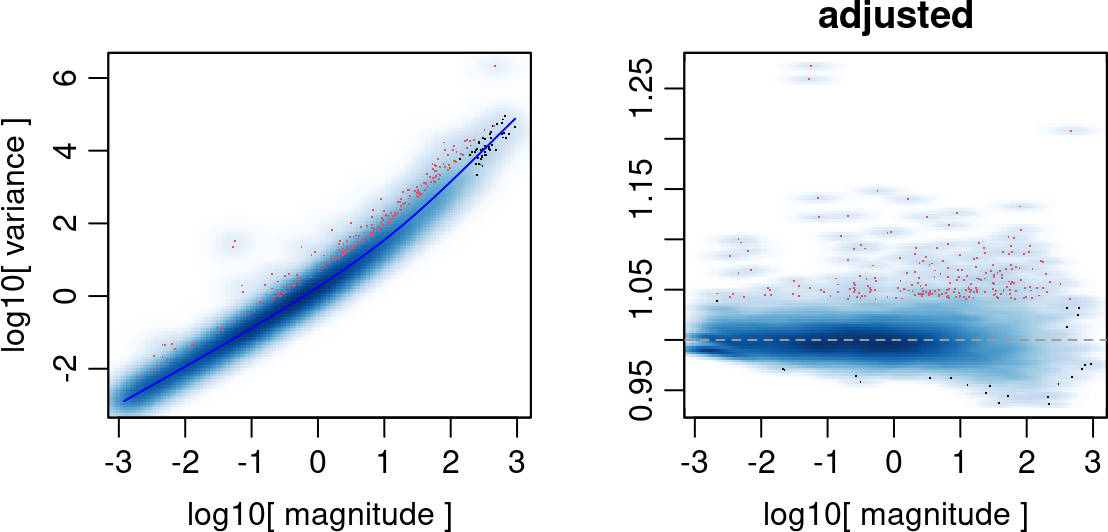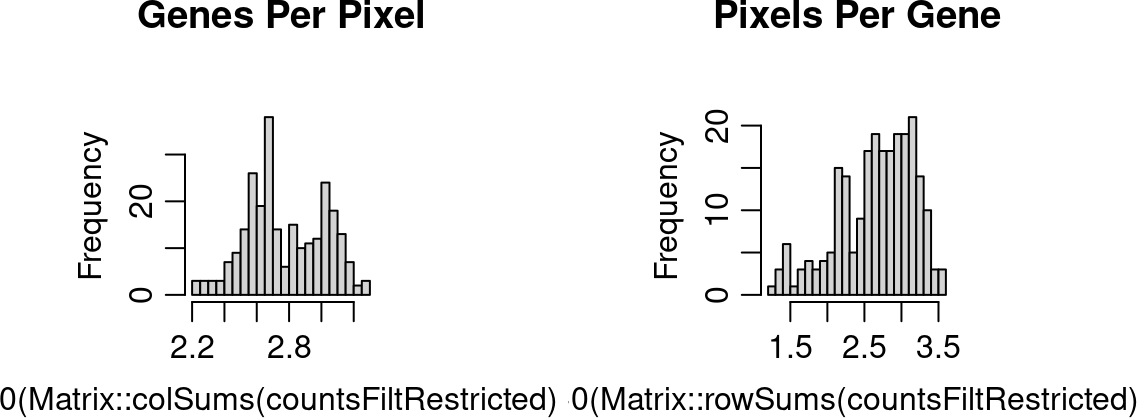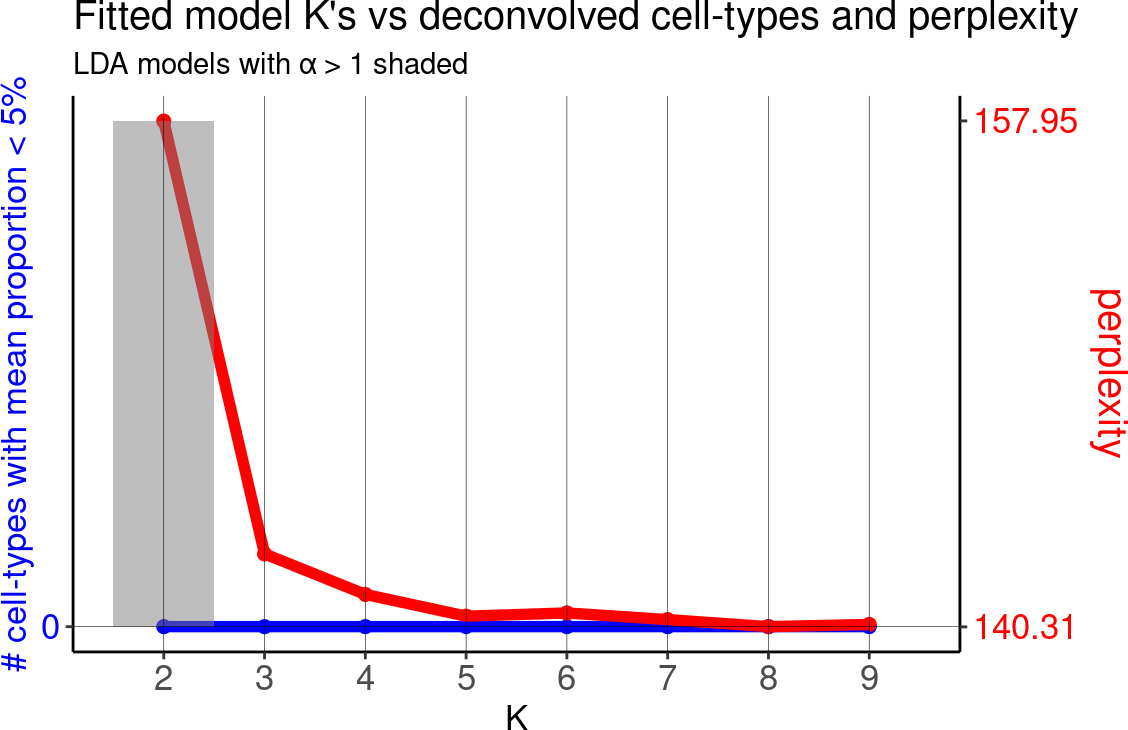# 1 Installation

``````if(!requireNamespace("BiocManager", quietly = TRUE))
install.packages("BiocManager")
BiocManager::install("STdeconvolve")``````

# 2 Introduction

`STdeconvolve` is an unsupervised machine learning approach to deconvolve multi-cellular pixel-resolution spatial transcriptomics datasets in order to recover the putative transcriptomic profiles of cell-types and their proportional representation within spatially resolved pixels without reliance on external single-cell transcriptomics references.

## 2.1 Deconvolution

In this tutorial, we will walk through some of the main functionalities of `STdeconvolve`.

``library(STdeconvolve)``

Given a counts matrix from pixel-resolution spatial transcriptomics data where each spatially resolved measurement may represent mixtures from potentially multiple cell-types, `STdeconvolve` infers the putative transcriptomic profiles of cell-types and their proportional representation within each multi-cellular spatially resolved pixel. Such a pixel-resolution spatial transcriptomics dataset of the mouse olfactory bulb is built in and can be loaded.

``````data(mOB)
pos <- mOB\$pos ## x and y positions of each pixel
cd <- mOB\$counts ## matrix of gene counts in each pixel
annot <- mOB\$annot ## annotated tissue layers assigned to each pixel``````

`STdeconvolve` first feature selects for genes most likely to be relevant for distinguishing between cell-types by looking for highly overdispersed genes across ST pixels. Pixels with too few genes or genes with too few reads can also be removed.

``````## remove pixels with too few genes
counts <- cleanCounts(counts = cd,
min.lib.size = 100,
min.detected = 1,
verbose = TRUE)``````
``## Converting to sparse matrix ...``
``## Filtering matrix with 262 cells and 15928 genes ...``
``## Resulting matrix has 260 cells and 14828 genes``
``````## feature select for genes
corpus <- restrictCorpus(counts,
removeAbove = 1.0,
removeBelow = 0.05,
alpha = 0.05,
plot = TRUE,
verbose = TRUE)``````
``## Removing 124 genes present in 100% or more of pixels...``
``## 14704 genes remaining...``
``## Removing 3009 genes present in 5% or less of pixels...``
``## 11695 genes remaining...``
``## Restricting to overdispersed genes with alpha = 0.05...``
``## Calculating variance fit ...``
``## Using gam with k=5...``
``## 232 overdispersed genes ...``
``##  Using top 1000 overdispersed genes.``
``##  number of top overdispersed genes available: 232```STdeconvolve` then applies latent Dirichlet allocation (LDA), a generative statistical model commonly used in natural language processing, to discover `K` latent cell-types. `STdeconvolve` fits a range of LDA models to inform the choice of an optimal `K`.

``````## Note: the input corpus needs to be an integer count matrix of pixels x genes
ldas <- fitLDA(t(as.matrix(corpus)), Ks = seq(2, 9, by = 1),
perc.rare.thresh = 0.05,
plot=TRUE,
verbose=TRUE)``````
``## Time to fit LDA models was 1.05 mins``
``## Computing perplexity for each fitted model...``
``## Time to compute perplexities was 0 mins``
``## Getting predicted cell-types at low proportions...``
``## Time to compute cell-types at low proportions was 0 mins``
``## Plotting...``In this example, we will use the model with the lowest model perplexity.

The shaded region indicates where a fitted model for a given K had an `alpha` > 1. `alpha` is an LDA parameter that is solved for during model fitting and corresponds to the shape parameter of a symmetric Dirichlet distribution. In the model, this Dirichlet distribution describes the cell-type proportions in the pixels. A symmetric Dirichlet with `alpha` > 1 would lead to more uniform cell-type distributions in the pixels and difficulty identifying distinct cell-types. Instead, we want models with `alpha` < 1, resulting in sparse distributions where only a few cell-types are represented in a given pixel.

The resulting `theta` matrix can be interpreted as the proportion of each deconvolved cell-type across each spatially resolved pixel. The resulting `beta` matrix can be interpreted as the putative gene expression profile for each deconvolved cell-type normalized to a library size of 1. This `beta` matrix can be scaled by a depth factor (ex. 1000) for interpretability.

``````## select model with minimum perplexity
optLDA <- optimalModel(models = ldas, opt = "min")

## Extract pixel cell-type proportions (theta) and cell-type gene expression
## profiles (beta) for the given dataset.
## We can also remove cell-types from pixels that contribute less than 5% of the
## pixel proportion and scale the deconvolved transcriptional profiles by 1000
results <- getBetaTheta(optLDA,
perc.filt = 0.05,
betaScale = 1000)``````
``## Filtering out cell-types in pixels that contribute less than 0.05 of the pixel proportion.``
``````deconProp <- results\$theta
deconGexp <- results\$beta``````

## 2.2 Visualization

We can now visualize the proportion of each deconvolved cell-type across the original spatially resolved pixels.

``````vizAllTopics(deconProp, pos,
groups = annot,
group_cols = rainbow(length(levels(annot))),
r=0.4)``````
``## Plotting scatterpies for 260 pixels with 8 cell-types...this could take a while if the dataset is large.``